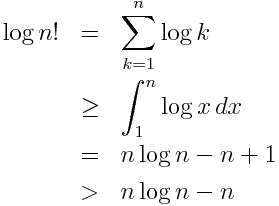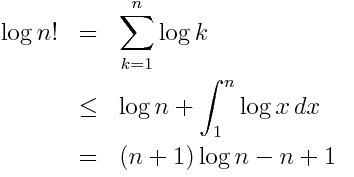# Simpler version of Stirling’s approximation

Here’s an approximation for n! similar to Stirling’s famous approximation.I ran into this reading A View from the Top by Alex Iosevich. It is less accurate than Stirling’s formula, but has three advantages.

1. It contains the highest order terms of Stirling’s formula.
2. It’s easy to derive.
3. It’s easy to remember.

One way to teach Stirling’s formula would be to teach this one first as a warm-up.

I’ll show that the approximation above gives a lower bound on n!. It gives a good approximation to the extent that the inequalities don’t give up too much.Exponentiating both sides showsThe derivation only uses techniques commonly taught in second-semester calculus classes: using integrals to estimate sums and integration by parts.

Deriving Stirling’s approximationrequires more work, but remembering the result is just a matter of adding an extra factor to the simpler approximation.

As I noted up front, the advantage of the approximation discussed here is simplicity, not accuracy. For more sophisticated approximation, see Two useful asymptotic series for how to compute the logarithm of the gamma function.

Update:

The first comment asked whether you tweak the derivation to get a simple upper bound on factorial. Yes you can.This leads to the upper boundWasteful compared to Stirling, but still simple to derive and remember.

## 6 thoughts on “Simpler version of Stirling’s approximation”

1. Alejandro Weinstein

David Mackay has an unconventional derivation of Stirling approximation. He approximate the Poisson distribution of parameter Lambda by a Gaussian of mean and variance Lambda (good approximation for big values of Lambda). The approximation follows from that.

See page 2 of his book “Information theory …”, or page 14 of the PDF, available here:

http://www.inference.phy.cam.ac.uk/mackay/itila/book.html

for the details.

2. SteveBrooklineMA

Nice. Can you tweak the derivation to get a simple upper bound?

3. Yes. The original derivation uses an integral to under-estimate the sum. You can shift the sum over by one and use an integral to over-estimate the sum.

Thanks for the suggestion. I’ve updated the post with an upper bound.